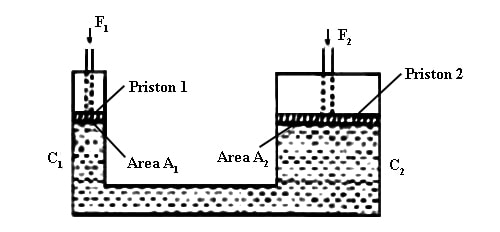# Explain Pascal’s Law

If the pressure is applied on any point of an enclosed liquid or gas it is transmitted in all directions. Pascal stated a law about this transmission of pressure.

Pascal’s law

External pressure applied to any portion of a liquid or a gas enclosed in a container is equally transmitted in all directions in the liquid or the gas without any trace of diminution and acts perpendicularly on the surface of the container in contact with the liquid or gas.Fig: Mathematical explanation of Pascal’s law

The mathematical explanation of Pascal’s law: the principle of multiplications of force

On any portion of a confined liquid if force is applied by a smaller piston, then forces of greater magnitudes are exerted on the pistons of greater cross-sectional area This is known as the principle of multiplication of force.

Let, C1 and C2 be two cylinders (Figure) and A1 and A2 be their cross-sectional areas respectively. The two cylinders are connected by a pipe. There is an airtight piston in each cylinder. The two cylinders are filled up with a liquid. Now a force F applied to the smaller piston generates a pressure F1/A1. According to Pascal’s law, this pressure is transmitted in all directions through the liquid. Therefore the upward pressure exerted on the larger piston is F1/A1. Because of this pressure, the larger piston experiences an upward force equal to (F1/A1) x A2. If the upward force of larger piston is F2,

Then, F2 = (F1/A1) x A2

So, (F2 / F1) = (A2 / A1)

So, greater is the cross-sectional area of the larger piston, the greater is the force exerted on it. If the cross-sectional area of the larger one is 100 times greater than that of the smaller one, then a force of 1N applied to the smaller one will produce an upward force of 100N on the larger piston.